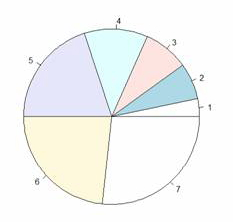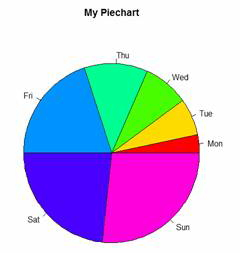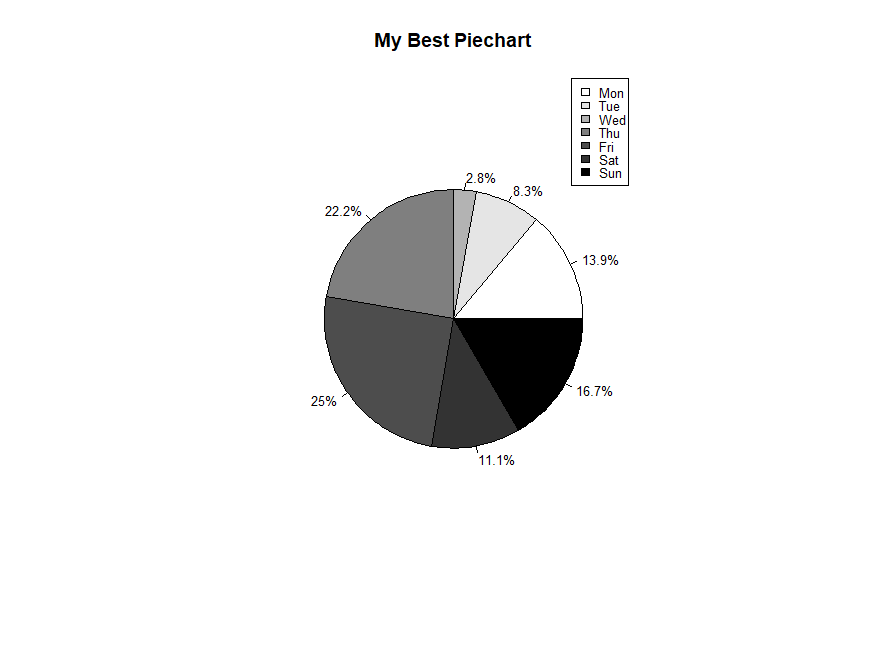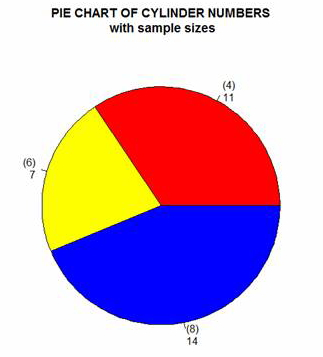# R Is Not So Hard! A Tutorial, Part 14: Pie ChartsIn Part 14, let’s see how to create pie charts in R. Let’s create a simple pie chart using the pie() command. As always, we set up a vector of numbers and then we plot them.

`B <- c(2, 4, 5, 7, 12, 14, 16)`

Create a simple pie chart.

`pie(B)`Now let’s create a pie chart with a heading, using nice colours, and define our own labels using R’s rainbow palette. We control the number of colours using length(B).

`B <- c(2, 4, 5, 7, 12, 14, 16)`
`pie(B, main="My Piechart", col=rainbow(length(B)),`
`labels=c("Mon","Tue","Wed","Thu","Fri","Sat","Sun"))`Here is a more complex example, using percentages and a legend. We create a vector of data, one for each day of the week

`B <- c(5, 3, 1, 8, 9, 4, 6)`

Set up black, grey and white for clear printing.

`cols <- c("white","grey90","grey70","grey50","grey30","grey20","black")`

Calculate the percentage for each day, using one decimal place.

`percentlabels<- round(100*B/sum(B), 1)`

Add a ‘%’ sign to each percentage value using the paste command.

`pielabels<- paste(percentlabels, "%", sep="")`

What does the paste command do?

`pie(B, main="My Best Piechart", col=cols, labels=pielabels, cex=0.8)`

Create a legend at the right.

`legend("topright", c("Mon","Tue","Wed","Thu","Fri","Sat","Sun"), cex=0.8, fill=cols)`OK. Now let’s create a pie chart from a data frame and include sample sizes. First create a table of counts of cylinder numbers from the mtcars data set.

`cyltable<- table(mtcars\$cyl)`
`cyltable`
`4 6 8`
`11 7 14`

We have eleven cars with four cylinders, seven cars with six cylinders, and fourteen cars with eight cylinders.

Now we create labels.

`labs<- paste("(",names(cyltable),")", "\n", cyltable, sep="")`

Now we plot.

```pie(cyltable, labels = labs, col = c("red", "yellow", "blue"), main="PIE CHART OF CYLINDER NUMBERS\n with sample sizes")```That wasn’t so hard! In Part 15 we will look at further plotting techniques in R.

About the Author: David Lillis has taught R to many researchers and statisticians. His company, Sigma Statistics and Research Limited, provides both on-line instruction and face-to-face workshops on R, and coding services in R. David holds a doctorate in applied statistics.Getting Started with R
Kim discusses the use of R statistical software for data manipulation, calculation, and graphical display.

1.Mark says

How do you get the labels inside the pie chart instead of outside?

2.Preethishetty says

Yeah, after reading this article I realized that it is easy to learn R. Thanks for posting such types of articles and motivating us to learn R Language.

3.Danielle says

How do you combine the names (labels) and the percentages (relative frequencies) on the graphs?

4.Gordon says

Awesome instruction. Real easy and effective to see how R works. I pasted your coding into Wordpad, cleaned out the notes, and pasted it straight into R and saw instantaneous results. Beautiful.

Please note that, due to the large number of comments submitted, any questions on problems related to a personal study/project will not be answered. We suggest joining Statistically Speaking, where you have access to a private forum and more resources 24/7.